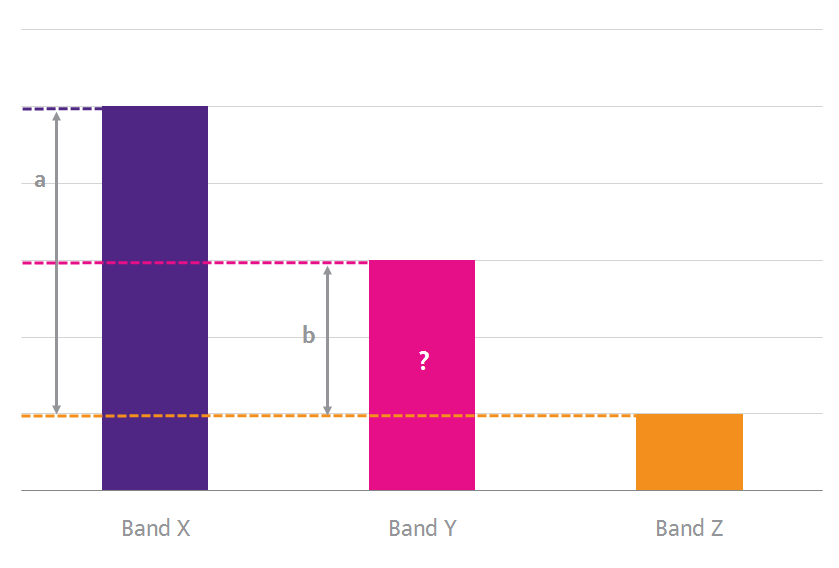Adjusted benchmarking is used in cases where there are few actual data points in a particular frequency band for direct benchmarking.  In this approach, past auction values for the target country will be important reference values. Adjusted benchmarking can be done using either relative value ratios or distance ratios.

In the relative value method, value ratios for the various frequency bands and band groupings are estimated from international benchmarks. There are many possible combinations and the value ratios that can be considered include for example:

• Sub-1 GHz to 1800 MHz and PCS
• Sub-1 GHz to AWS and 2100 MHz
• Sub-1 GHz to 2.5 GHz values
• 1800 MHz and PCS to 2.5 GHz
• AWS and 2100 MHz to 2.5 GHz

These ratios are then applied to the appropriate past auction results in the target country to estimate the relevant values for the different bands.

The distance method makes use of observed distance ratios of selected reference bands relative to the band to be valued as shown in the figure below.  In this example the objective is to estimate a value for band Y in Country 1 using the values of bands X and Z from previous auctions as reference points. The distance ratio is derived from appropriate benchmark countries for which the values of all three bands (X, Y, Z) are available.  This ratio (a/b) is then applied to calculate the value of band Y in Country 1 using its values for band X and band Z.